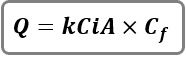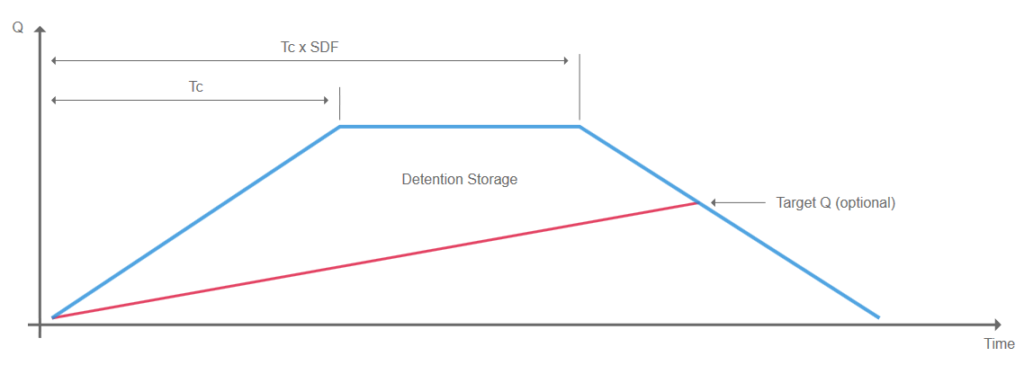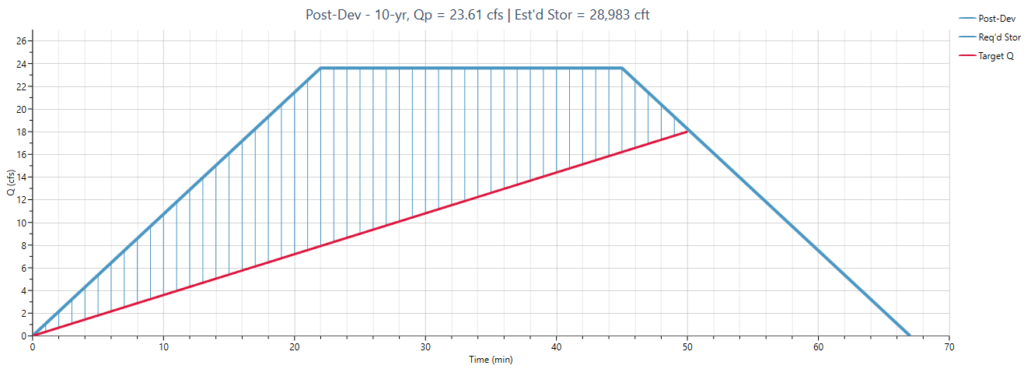1. Home
2. Computational Methods
3. Rational Method Hydrographs

# Rational Method Hydrographs

The Rational method was developed over 100 years ago and continues to be used for urban watershed modeling, typically on areas less than about 20 acres. Studio Express can generate two kinds of Rational Method hydrographs;

1. Standard Rational
2. Modified Rational

## Standard Rational

The Standard Rational Method hydrograph is shaped like an isosceles triangle. The Peak is equivalent to the peak discharge as determined by the well known Rational formula.Where:
Qp = hydrograph peak discharge (cfs)
K = 1.0 (0.00278 metric)
C = runoff coefficient
A = basin area (ac)
i = intensity (in/hr)
Cf = frequency correction factor

The time-to-peak of the hydrograph is equal to the time of concentration. The hydrograph is an isosceles triangle. Intermediate hydrograph values are computed using straight-line interpolation.

## Modified Rational

This method modifies the Standard Rational method. The Modified Rational Method uses the peak flow calculating capability of the Rational Method paired with assumptions about the inflow and outflow hydrographs to compute an approximation of storage volumes for simple detention calculations.

The runoff hydrograph is assumed to be trapezoidal in shape with a peak runoff rate calculated using the rational formula described above. Studio Express finds the Storm Duration Factor (SDF) which maximizes the required storage of an anticipated detention pond routing. In other words, the rising and falling limbs of the inflow hydrograph have a duration equal to the time of concentration, Tc. A target outflow is set (Qt) based on pre-development conditions. The storm duration is increased until the storage volume (blue shaded area) is maximized.Updated on May 9, 2018